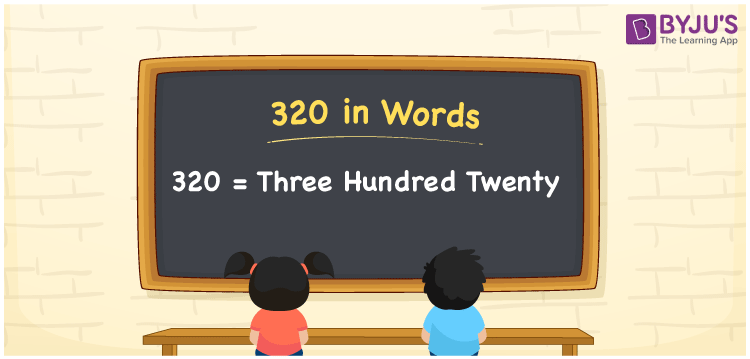# 320 in Words

320 in words can be written as Three Hundred Twenty. The concepts like counting numbers which are important from primary education are discussed here in brief. For instance, if you spend Rs. 320 in buying a pant, then you can say that “I bought a pant for Three Hundred Twenty Rupees”. The English alphabet is used to write 320 in words. Students can get a clear knowledge of numbers in words with the help of the study materials given at BYJU’S. The number 320 can be read as “Three Hundred Twenty” in English.

 320 in words Three Hundred Twenty Three Hundred Twenty in Numbers 320

## 320 in English Words## How to Write 320 in Words?

Place value chart and expanded form of 320 can be learnt easily in this section. There are three digits in the number 320. The place value chart for 320 is provided below for reference to help students understand the concepts effectively.

 Hundreds Tens Ones 3 2 0

The expanded form of 320 is provided below:

3 × Hundred + 2 × Ten + 0 × One

= 3 × 100 + 2 × 10 + 0 × 1

= 320

= Three Hundred Twenty

Hence, 320 in words is written as Three Hundred Twenty.

320 is a natural number that precedes 321 and succeeds 319.

320 in words – Three Hundred Twenty

Is 320 an odd number? – No

Is 320 an even number? – Yes

Is 320 a perfect square number? – No

Is 320 a perfect cube number? – No

Is 320 a prime number? – No

Is 320 a composite number? – Yes

## Frequently Asked Questions on 320 in Words

### How can 320 be written in words?

320 can be written as “Three Hundred Twenty” in words.

### Is 320 an odd number?

No, 320 is not an odd number because it is divisible by 2.
320/2 = 160

### Write Three Hundred Twenty in numbers.

Three Hundred Twenty can be written in numbers as 320.